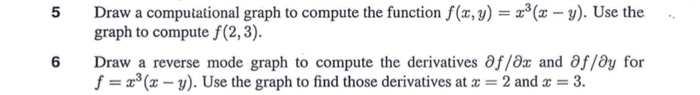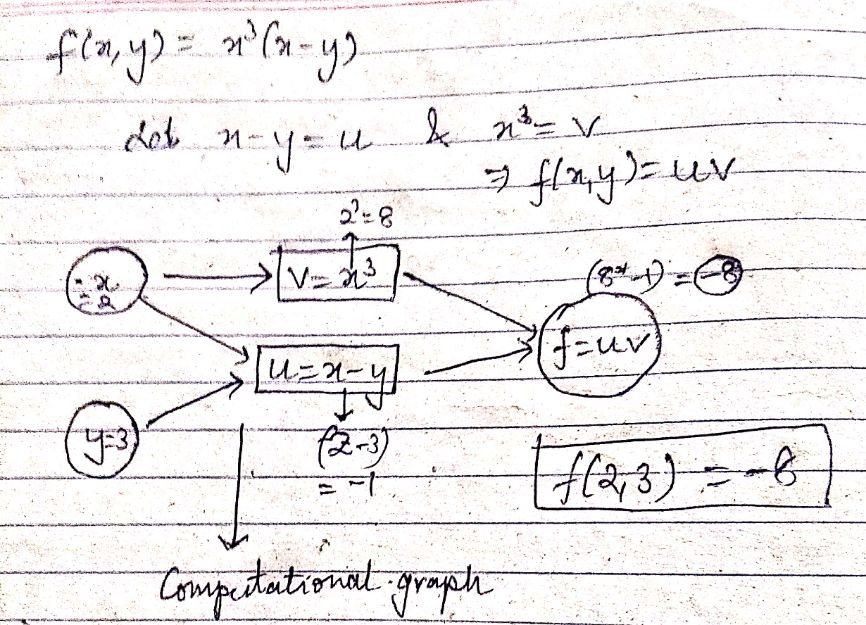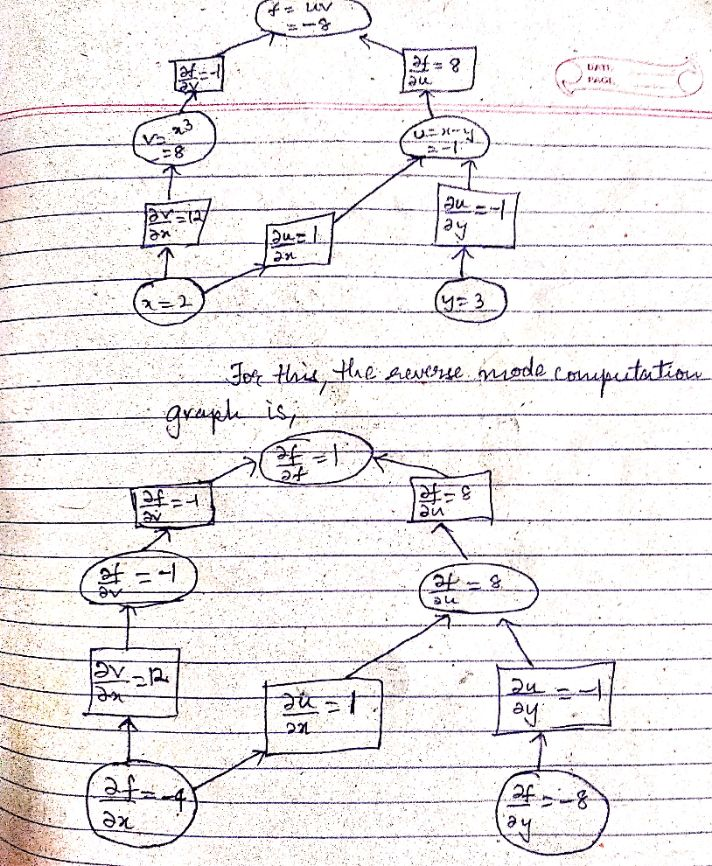# 5 Draw a computational graph to compute the function f(, y) ( graph to compute f (2,3) -y). Use the Draw a reverse mode graph to compute the derivatives f/0x and อ//ay for f 3( y). Use the graph...5 Draw a computational graph to compute the function f(, y) ( graph to compute f (2,3) -y). Use the Draw a reverse mode graph to compute the derivatives f/0x and อ//ay for f 3( y). Use the graph to find those derivatives at 2 and 3. 6Consider the equationTo draw the computational graph, we simplify the equation into it's constituent terms i.e.,

LetSo,For the reverse-mode graph, first we compute the partial derivatives :##### Add Answer of: 5 Draw a computational graph to compute the function f(, y) ( graph to compute f (2,3) -y). Use the Draw a reverse mode graph to compute the derivatives f/0x and อ//ay for f 3( y). Use the graph...
More Homework Help Questions Additional questions in this topic.